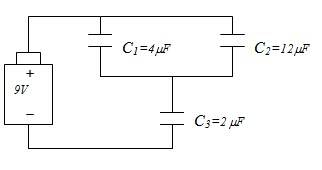# Charge on and the potential difference across capacitors

## Homework Statement

Calculate the charge on and the potential difference across each capacitor## Homework Equations

C|| = C1+C2+C3...
C(Series) [(1/C1)+(1/C2)+(1/C3)+...]$^{-1}$
Q=CV
V=Q/C

## The Attempt at a Solution

I already know that C$_{123}$ = 16/9 $\mu$F

I also already know that the Net Charge Q = 16 $\mu$C

My actual attempt at the solutions is that Q$_{1}$=Q$_{2}$=Q$_{3}$=Q$_{net}$=16 $\mu$C

I also got that V3=16/2= 8 V, V1=16/4= 4 V, and V2=16/12= 1.3 V

Is this correct? Or did I mess up somewhere? This is on paper submission so I cannot check if it is correct or not until the TAs grade it.

gneill
Mentor
The charges on all three capacitors won't all be the same.

Series connected capacitors will have the same net charge. So the parallel combination of C1 and C2 will have the same charge as C3.

Your net charge calculation, Q = 16 μC, is good. So C3 will have 16 μC on it. You should be able to work out the voltage across C3 from that. The remaining voltage (to add up to the battery voltage) must then be across C1 and C2 which are in parallel. You should be able to find that remaining voltage and then using it, the charges on C1 and C2.

Wow, that was very helpful. I was fiddling with some other solutions and one was in that direction but when I hit the remaining voltage for C1 and C2 for 1V, I discarded it because I thought it was wrong.

So I have 1 V to split between C1 and C2

so C1 has a charge of 4 μC and C2 has a charge of 12 μC, if I understand you correctly?

gneill
Mentor
Wow, that was very helpful. I was fiddling with some other solutions and one was in that direction but when I hit the remaining voltage for C1 and C2 for 1V, I discarded it because I thought it was wrong.

So I have 1 V to split between C1 and C2

so C1 has a charge of 4 μC and C2 has a charge of 12 μC, if I understand you correctly?

You understand correctly